date: “2022-11-16” output: rmarkdown::html_vignette vignette: > % % % —

## Introduction

The smacpod package implements methods for analyzing case-control point pattern data. It relies on the spatstat package for many of the internal computations, and many function arguments are simply the relevant arguments of a spatstat function.

We briefly summarize functionality below using the grave data set. The grave data set provides information related to 143 medieval grave locations. 30 of the graves are "affected" by a tooth deformity, while the remaining graves are "unaffected" by the deformity. The grave data set is a ppp object, which is a class utilized by the spatstat package.

We load and plot the data to start.

# load packages
library(smacpod)
data(grave)
# pch changes point symbol
plot(grave, pch = c(1, 19), main = "grave locations", xlab = "x", ylab = "y")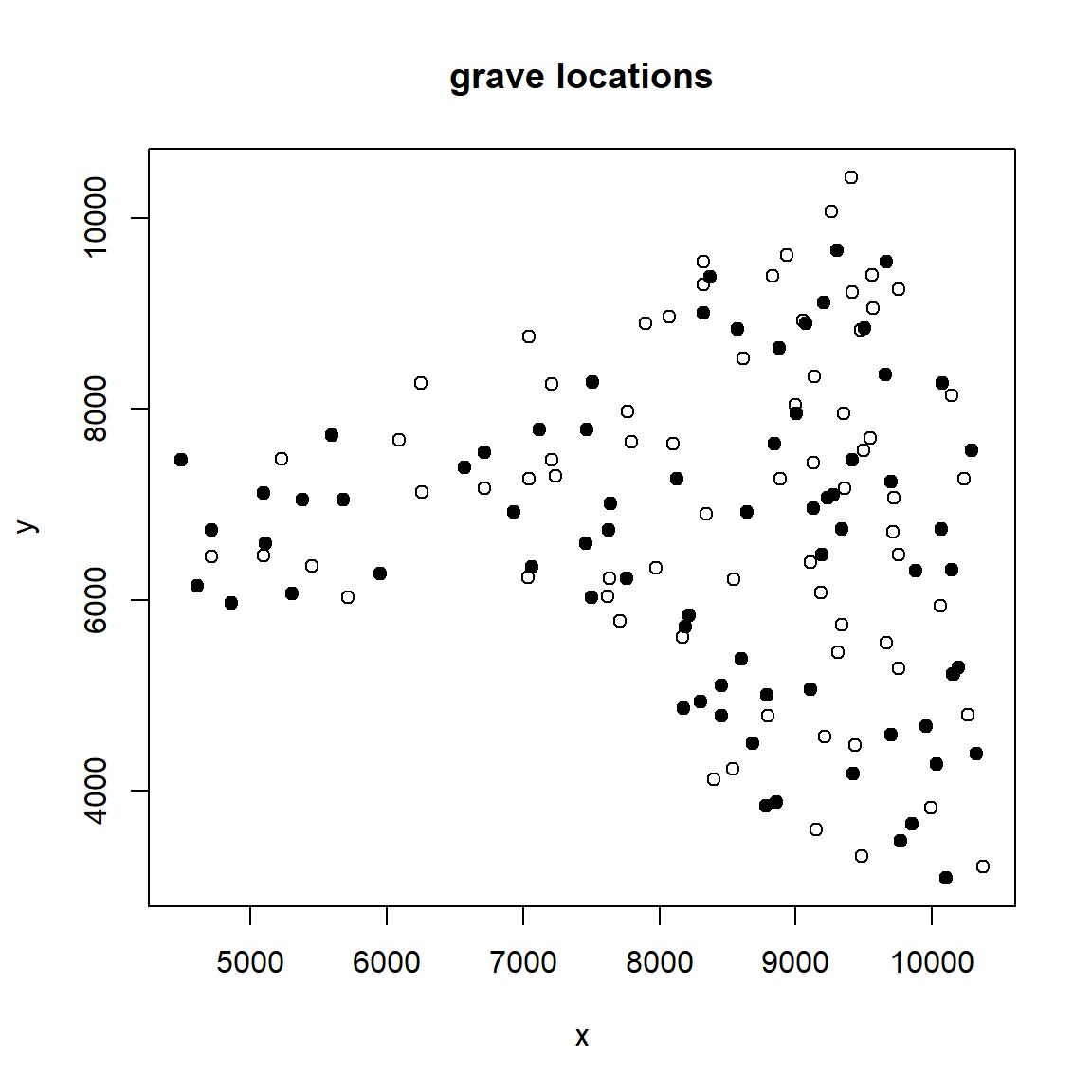## Spatial density

The spdensity function computes the spatial density function of a point pattern. The spatstat package provides a density function, which is actually the estimated intensity of a point pattern. The spdensity function scales the intensity to integrate to 1. The result is an im object, a class from the spatstat package, which has an associated plot method. We estimate and plot the spatial density of the affected grave locations below. We do not specify the bandwidth or kernel function used in the estimation process, so the default choices of the spatstat package are used. The heat map of the estimated spatial density indicates greater density of grave locations in the lower right part of the study area.

# identify affected observations
aff <- (grave$marks == "affected") # estimated spatial density function for affected observations f <- spdensity(grave[aff,]) # plot spatial density function of affected plot(f, "spatial density of affected graves")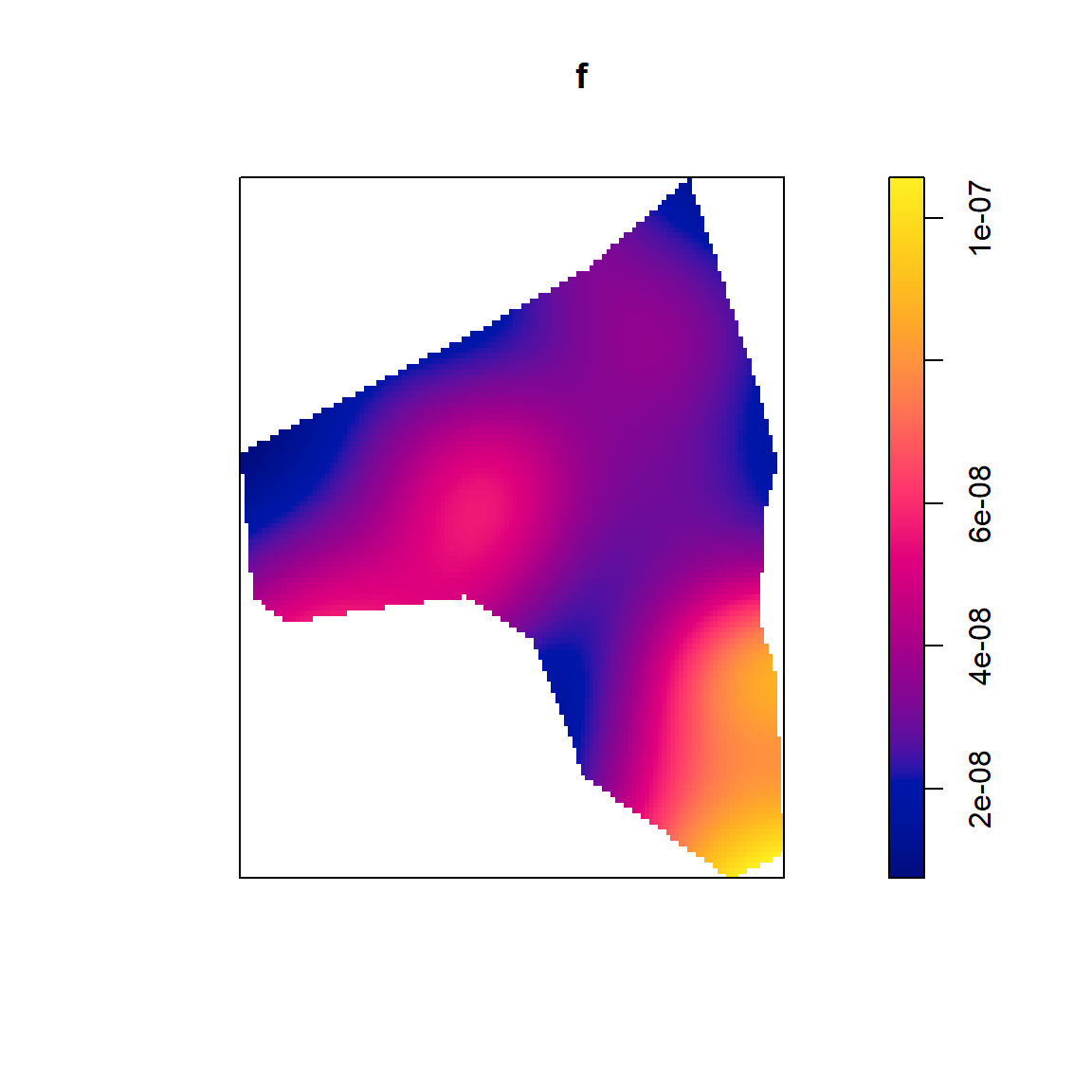# Log relative risk Let $$D$$ denote the study area under consideration. Let $$\lambda_1(s)$$ denote the intensity function at location $$s$$ of the group designated as the case group. Then the spatial density of the case group can be denoted as $f(s)=\frac{\lambda_1(s)}{\int_D \lambda_1(s) ds}.$ Similarly the intensity function and spatial denstiy function of the control group are denoted as $$\lambda_0(s)$$ and $$g(s)$$, respectively. The log relative risk function is defined as $r(s) = \ln\left(\frac{f(s)}{g(s)}\right).$ The log relative risk function can be estimated by $\hat{r}(s) = \ln\left(\frac{\hat{f}(s)}{\hat{g}(s)}\right),$ where $$\hat{f}$$ and $$\hat{g}$$ are the estimates of $$f$$ and $$g$$ from the spdensity function. The log relative risk can be estimated using the logrr function. The function takes: • x: a ppp object with marks for the cases and controls • sigma: the bandwidth parameter of the case group • sigmacon: the bandwidth parameter of the control group • case: a character string specifying the name of the case group or the index of the case group in levels(x$marks). The default is case = 2, the second level of x$marks. If sigma and sigmacon aren’t specified, then the spatstat.explore::bw.relrisk function is used for these arguments. We estimate the log relative risk below for the grave data, selecting the affected graves as the case group, and then plot the results. The logrr returns an object of class im, which has an associated plot method. We also add contours of $$\hat{r}(s)$$ using the contour function. The ares with levels around 1-1.5 have the highest relative risk of cases to controls. These are the areas most likely to have a cluster of affected grave locations relative to unaffected grave locations. rhat <- logrr(grave, case = "affected") ## affected has been selected as the case group plot(rhat, main = "log relative risk of affected versus unaffected graves") contour(rhat, add = TRUE)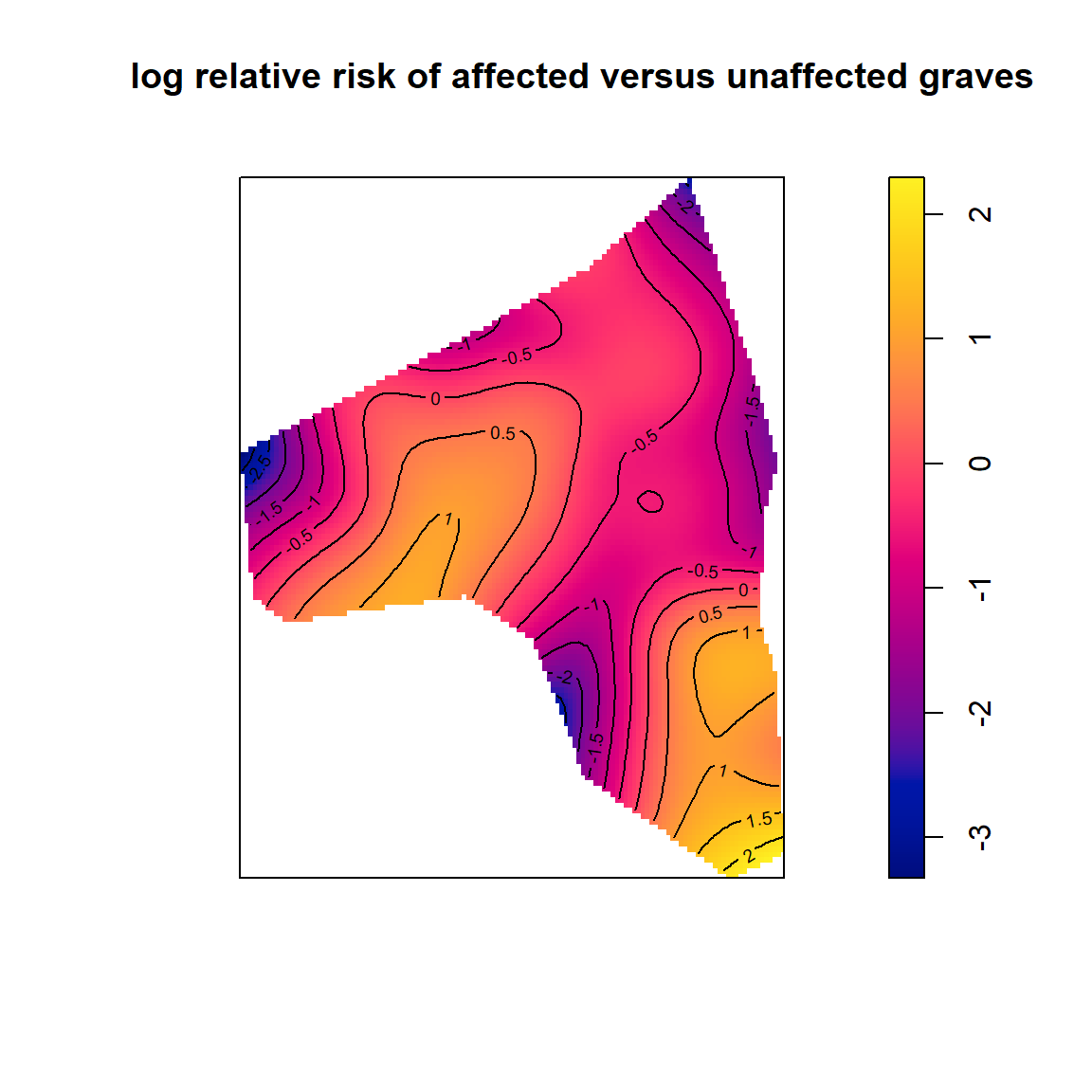If the ratio $$f(s)/g(s)$$ is constant, then the relative risk is 1 and $$r(s)=0$$. To determine where the log relative risk differs significantly from zero, we can simulate nsim new case-control data sets under the random labeling hypothesis, estimate $$r(s)$$ for each simulated data set, and construct tolerance envelopes for $$r(s)$$ at each location. If $$\hat{r}(s)$$ is outside the tolerance envelopes, then the estimated log relative risk is inconsistent with $$r(s)=0$$ under the random labeling hypothesis. This is the same is believing the spatial densities of the cases and controls differ from zero at that location. If the spatial density of the cases is greater than the spatial density of the controls, then we believe there is a cluster of cases relative to the controls. If the spatial density of the controls is greater than the spatial density of the cases, then we believe there is a cluster of controls relative to the cases. The tolerance envelopes can be constructed by specifying the following arguments of logrr: • nsim: the number of randomly labeled data sets to generate. • level: the level of the tolerance envelopes. The default is 0.90. • alternative: the type of envelopes to construct. The default is two.sided. When nsim>0, the logrr function returns an object of class renv, which has a plot method that makes it easy to identify the parts of the study area where the estimated log relative risk is outside the tolerance envelopes. Any colored area indicates a location where the log relative risk is outside the tolerance envelopes. Non-colored areas indicate the estimated log relative risk is inside the tolerance envelopes. Similarly, there is a print method for the renv class summarizing information about the object constructed. For the grave data, the yellow areas indicate parts of the study area where the affected grave locations are clustered relative to the unaffected grave locations beyond what is expected under the random labeling hypothesis. The purple areas indicate parts of the study area where the unaffected grave locations are clustered relative to the affected grave locations beyond what is expected under the random labeling hypothesis. # construct tolerance envelopes for logrr renv = logrr(grave, case = "affected", nsim = 99, level = 0.90) ## affected has been selected as the case group # print info for tolerance envelopes renv ## ## pixelwise tolerance envelopes for log relative risk, r(s) ## ## r(s) = ln[f(s)/g(s)] ## f(s) = spatial density of cases at location s ## g(s) = spatial density of controls at location s ## case label: affected ## control label: unaffected ## number of simulations: 99 ## simulation procedure: random labeling ## envelope level: 0.9 # plot areas with rhat outside of envelopes plot(renv)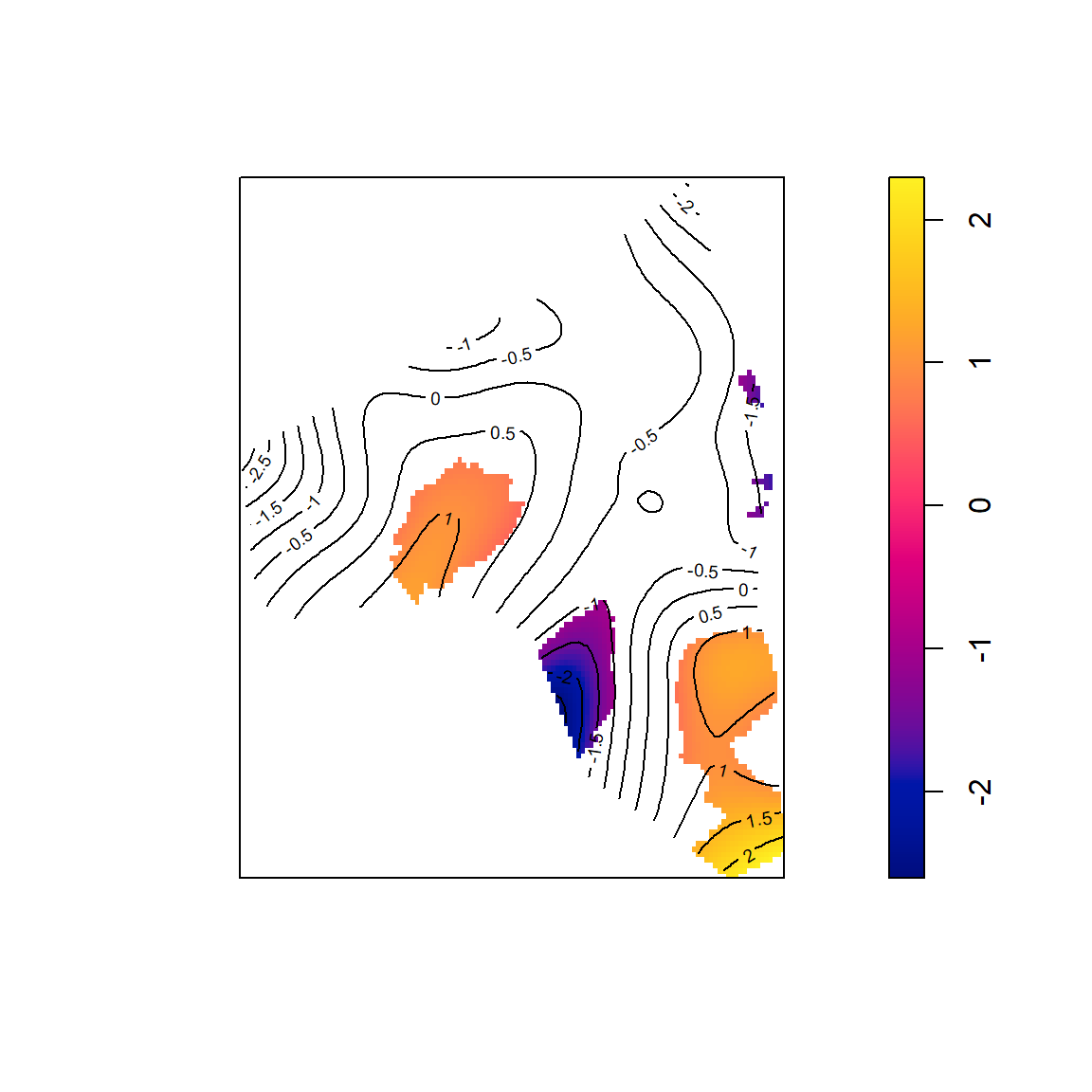The color scale can be manipulated to use different colors, as in the example below. # a better color scale (in my opinion) # making it easier to distinguish the clusters of cases relative # to controls (red) and vice versa (blue) grad = gradient.color.scale(min(renv$v, na.rm = TRUE), max(renv$v, na.rm = TRUE)) plot(renv, col = grad$col, breaks = grad$breaks)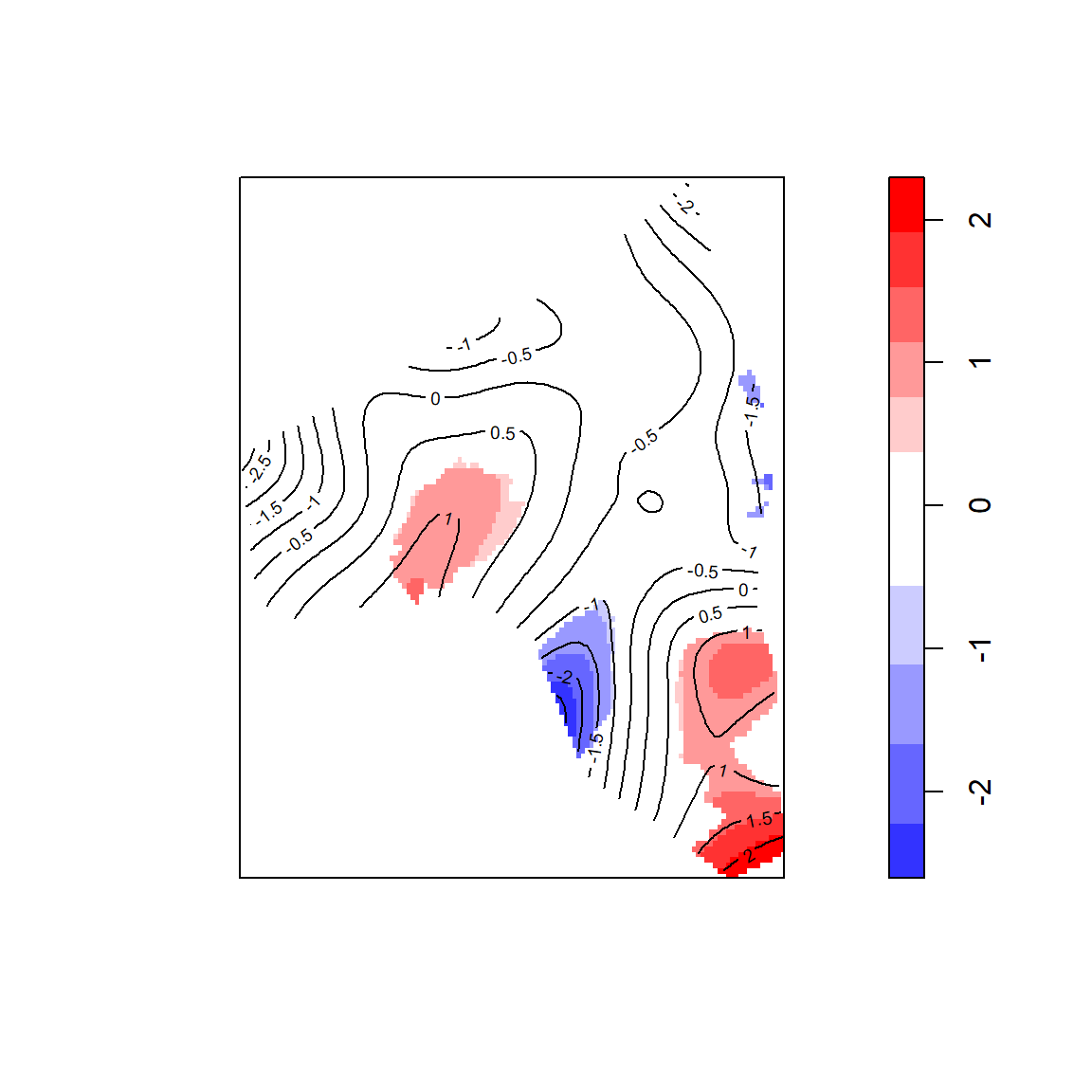Because the tolerance envelopes do not account for multiple comparisons, Kelsall and Diggle (1995) proposed a test of $$H_0: r(s) = 0$$ for all $$s$$ in $$D$$ $$H_a: r(s) \neq 0$$ for at least one $$s$$ in $$D$$ using the test statistic $$\int_D \{\hat{r}(s)\}^2\,ds$$. This test is implemented in the logrr.test function, which takes an object of class renv. For the grave data, since the p-value is relative large, there isn’t convincing evidence that the log relative risk of the affected and unaffected grave locations differs from 0 for at least one location in the study area. logrr.test(renv) ## ## Kelsall and Diggle (1995) test for log relative risk ## ## r(s) = ln[f(s)/g(s)] ## f(s) = spatial density of cases at location s ## g(s) = spatial density of controls at location s ## case label: affected ## control label: unaffected ## ## null hypothesis: r(s) = 0 for all s in study area ## alternative hypothesis: r(s) != 0 for at least one s in study area ## test statistic: 19478034 ## p-value: 0.22 ## nsim: 99 ## simulation procedure: random labeling ## Difference in K functions Ripley’s K function is often used to assess the variability of the distances between events for point patterns. A difference in K functions can be used to compare the clustering behavior for case-control point data, where $\widehat{KD}(r) = \widehat{K}_{case}(r) - \widehat{KD}_{control}(r)$ is the estimated difference in K functions for the case and control groups for a specific distance $$r$$. The kdest function can be used to compute $$\widehat{KD}(r)$$. The function requires: • x: a ppp object with marks for the cases and controls • case: a character string specifying the name of the case group or the index of the case group in levels(x$marks). The default is case = 2, the second level of x$marks. The function relies internally on spatstat.explore::Kest, and users are directed there for more details about the estimation process. The kest function produces on object of class fv, defined by the spatstat.explore package, which has an associated plot method that can be used to plot the results. We compute the estimated difference in K functions for the case and controls groups below, and then plot the results. kd = kdest(grave, case = "affected") ## affected has been selected as the case group plot(kd, cbind(iso, theo) ~ r, legend = FALSE, main = "")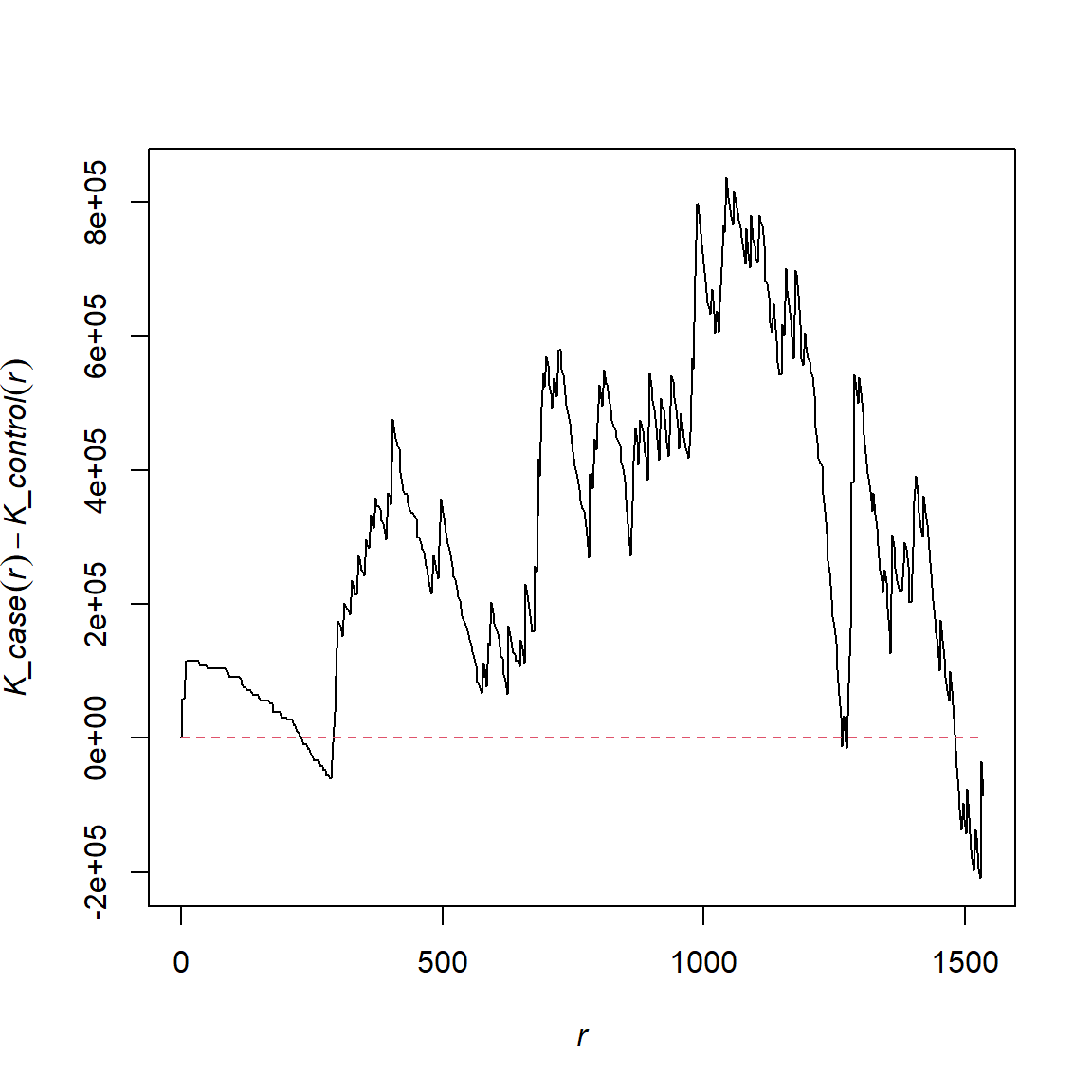To investigate where $$\widehat{KD}(r)$$ differs significantly from zero, tolerance envelopes for $$KD(r)$$ can be estimated by simulating data under the random labeling hypothesis. We need to specify: • nsim: the number of randomly labeled data sets to generate. • level: the level of the tolerance envelopes. The default is 0.90. When nsim>0, the kdest function returns an object of class kdenv. print, summary, and plot methods are defined for kdenv objects. The print method will print information about the kdenv object, summary will summarize the distances at which $$\widehat{KD}(r)$$ is outside the tolerance envelopes, and plot will plot $$\widehat{KD}(r)$$ versus $$r$$, along with the average $$\widehat{KD}(r)$$ for data sets simulated under the null hypothesis, the tolerance envelopes, and envelopes of the minimum and maximum $$\widehat{KD}(r)$$ for the simulated data sets. We contruct tolerance envelopes for $$KD(r)$$ based on 49 data sets simulated under the random labeling hypothesis at the 0.95 level. Using the output of the summary function, we see that $$\widehat{KD}(r)$$ is outside the tolerance envelopes for $$r$$ in the (approximate) intervals, 9-48, 353-387, 395-425, 985-1004, 1081-1085, and at $$r=1090.454$$. The corresponding plot is shown below with a manual legend created to identify the various parts of the plot. kdenv <- kdest(grave, case = "affected", nsim = 49, level = 0.95) ## affected has been selected as the case group ## Generating 49 simulations by evaluating expression ... ## 1, 2, 3, 4, 5, 6, 7, 8, 9, 10, 11, 12, 13, 14, 15, 16, 17, 18, 19, 20, 21, 22, 23, 24, 25, 26, 27, 28, 29, 30, 31, 32, 33, 34, 35, 36, 37, 38, 39, 40, ## 41, 42, 43, 44, 45, 46, 47, 48, 49. ## ## Done. # print some information about kdenv print(kdenv) ## ## Envelopes for difference in estimated K functions ## ## KD(r) = K_case(r) - K_control(r) ## case label: affected ## control label: unaffected ## KD(r) computed for r betwen 0 and 1533.825 ## number of simulations: 49 ## simulation procedure: random labeling ## envelope level: 0.95 # determine distances KD(r) outside tolerance envelopes summary(kdenv) ## KD(r) > upper envelope limit for the following r: ## 8.987257 to 47.93204 ## 353.4988 to 386.4521 ## 395.4393 to 425.3968 ## 985.6025 to 1003.577 ## 1081.467 to 1084.462 ## 1090.454 # plot results plot(kdenv, ylab = "difference in K functions") legend("topleft", legend = c("obs", "avg", "max/min env", "95% env"), lty = c(1, 2, 1, 2), col = c("black", "red", "darkgrey", "lightgrey"), lwd = c(1, 1, 10, 10))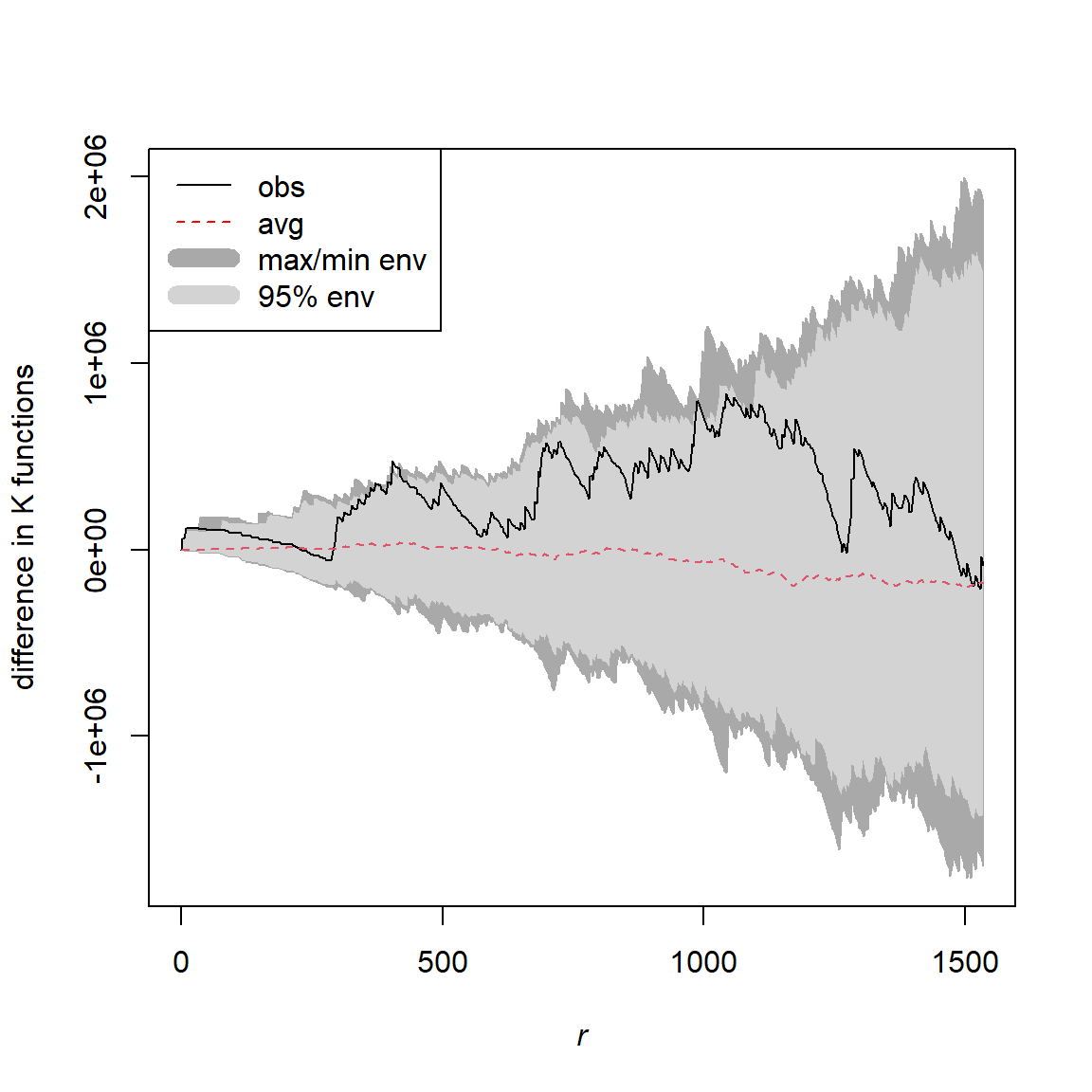The tolerance envelopes do not account for multiple comparisons, so Diggle and Chetwynd (1991) proposed a test of $$H_0: KD(r) = 0$$ for all $$r$$ in $$[0, r_{max}]$$ $$H_a: KD(r) > 0$$ for at least one $$r$$ in $$[0, r_{max}]$$ using the test statistic $$KD_+=\sum_{i=1}^m \widehat{KD}(r_i)$$, where $$r_1,\ldots,r_m$$ are the sequence of values for which $$\widehat{KD}(r)$$ is estimated. This test is implemented in the kdplus.test function. For the grave data, since the p-value is somewhat small (0.06), there is weak evidence that the affected grave locations are clustered relative to the unaffected grave locations for some distance between 0 and 1533 meters. kdplus.test(kdenv) ## ## Diggle and Chetwynd (1991) test for difference in K functions ## ## KD(r) = K_case(r) - K_control(r) ## case label: affected ## control label: unaffected ## ## null hypothesis: KD(r) = 0 for all r between 0 and 1533.825 ## alternative hypothesis: KD(r) > 0 for at least one r between 0 and 1533.825 ## test statistic: 576.2432 ## p-value: 0.06 ## nsim: 49 ## simulation procedure: random labeling ## Spatial scan test Kulldorff (1997) proposed a spatial scan test for case-control point data. The test scans the study area using circular windows to identify windows with unusual collections of cases compared to what is outside the window. The significance of the most likely cluster (window) is assessed via the random labeling hypothesis. The test is implemented using the spscan.test function, which takes the arguments: • x: a ppp object with marks for the cases and controls • case: a character string specifying the name of the case group or the index of the case group in levels(x$marks). The default is case = 2, the second level of x$marks. • nsim: the number of randomly labeled data sets to generate. • alpha: the significance level of the test • maxd: the maximum size of the window. the default is half the maximum inter-event distance. The method assess whether the most likely cluster of cases is significant under the random labeling hypothesis. It can also be used to identify the most likely clusters of events that do not overlap. The print function will summarize some basic information of the test. The summary function will summarize any identified clusters. The clusters function will extract the events comprising the significant clusters. The plot function will draw the most likely and any secondary clusters. For the grave data, we identify one significant cluster. The cluster is centered at $$(10324, 4389)$$ with a radius of 1359.7 meters and includes 19 events (11 affected graves). The cluster was found on the lower right part of the study area. The cluster includes events 126, 124, …, 75. scan = spscan.test(grave, nsim = 49, case = "affected") ## affected has been selected as the case group # print info about scan scan ## method: circular scan ## distance upperbound: 3689.4017468961 ## realizations: 49 # summary of scan test results summary(scan) ## centroid_x centroid_y radius events cases ex rr stat p ## 1 10324 4389 1359.7 19 11 4 3.8 7.4 0.1 # plot scan test results plot(scan, chars = c(1, 20), main = "most likely cluster for grave data", border = "orange")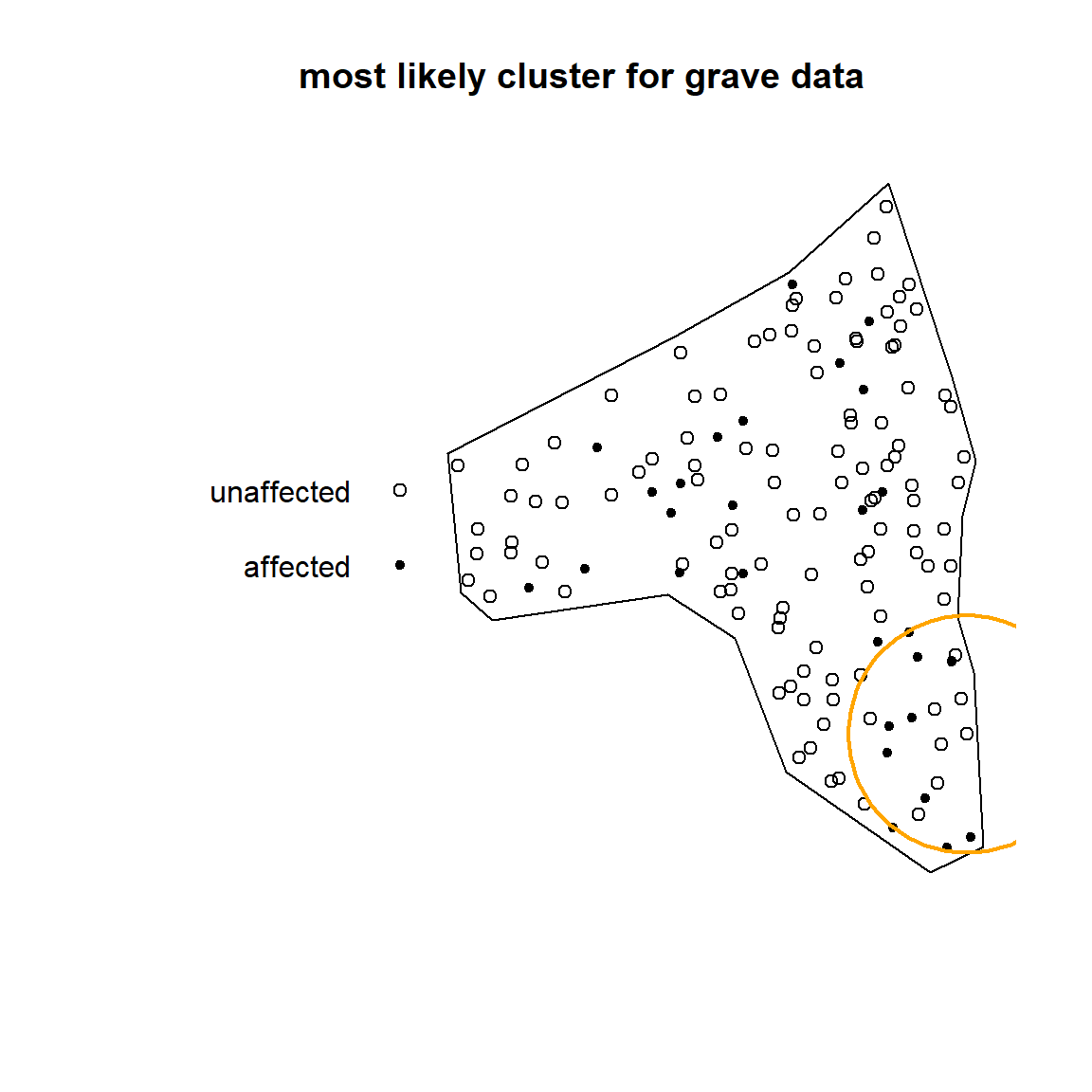# extract most likely and other significant clusters clusters(scan) ## [] ##  126 124 125 132 120 113 140 141 112 41 136 30 133 108 31 143 110 139 75 ## Q nearest neigbors Cuzick and Edwards (1990) proposed a test to examine whether the number of cases within the $$Q$$ nearest neighbors of other cases is unusually large relative to the random labeling hypothesis. This test is implemented in the qnn.test function, which takes the arguments: • x: a ppp object with marks for the cases and controls • q: a sequence of $$Q$$ values to consider • case: a character string specifying the name of the case group or the index of the case group in levels(x$marks). The default is case = 2, the second level of x\$marks.
• nsim: the number of randomly labeled data sets to generate.

Note that the statistics for different $$Q$$ will be correlated since, for example, the 3 nearest neighbors of a case will be included in the 5 nearest neighbors of a case. Thus, the test also measures the significance of the excess number of cases for different values of $$Q$$, i.e., the contrast between the test statistic for different values of $$Q$$. If the contrast is not significant, then any clustering observed is caused by the clustering observed for the smaller value of $$Q$$.

For the grave data, we examine whether there is excess clustering of cases among the $$Q$$ nearest neighbors of each case for $$Q=3,5,\ldots,15$$, using the affected group as the case group. Since the p-value is small for each value of $$Q$$, there is evidence of a significant excess of cases among the $$Q$$ nearest neighbors of each case compared to the random labeling hypothesis for $$Q=3,5,\ldots,15$$. Looking at the p-values for the contrasts, it appears that the clustering of cases observed for $$Q=5,7,9,11$$ are caused by the $$Q=3$$ nearest neighbors. However, the clustering observed at $$Q=13,15$$ is in excess to the clustering observed at $$Q=3$$. Similarly, the clustering observed for $$Q=15$$ is caused by the clustering observed for $$Q=13$$.

qnn.test(grave, q = c(3, 5, 7, 9, 11, 13, 15), nsim = 49, case = "affected")
## affected has been selected as the case group
## Q nearest neighbors test
##
## case label:  affected
## control label:  unaffected
##
## Summary of observed test statistics
##
##   q  Tq pvalue
##   3  32   0.02
##   5  45   0.04
##   7  58   0.04
##   9  73   0.04
##  11  91   0.04
##  13 109   0.02
##  15 122   0.06
##
## Summary of observed contrasts between test statistics
##
##   contrast Tcontrast pvalue
##    T5 - T3        13   0.54
##    T7 - T3        26   0.46
##    T9 - T3        41   0.26
##   T11 - T3        59   0.16
##   T13 - T3        77   0.14
##   T15 - T3        90   0.12
##    T7 - T5        13   0.42
##    T9 - T5        28   0.28
##   T11 - T5        46   0.14
##   T13 - T5        64   0.10
##   T15 - T5        77   0.12
##    T9 - T7        15   0.20
##   T11 - T7        33   0.08
##   T13 - T7        51   0.08
##   T15 - T7        64   0.10
##   T11 - T9        18   0.10
##   T13 - T9        36   0.06
##   T15 - T9        49   0.10
##  T13 - T11        18   0.08
##  T15 - T11        31   0.16
##  T15 - T13        13   0.46